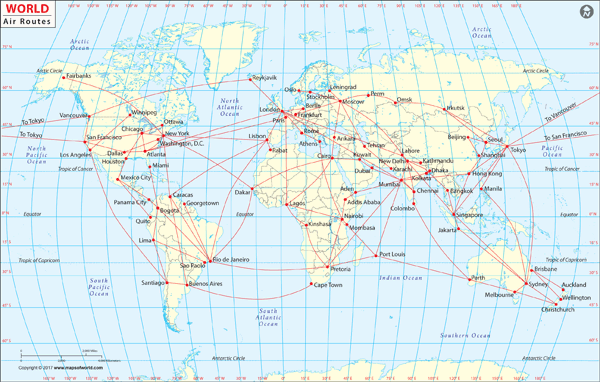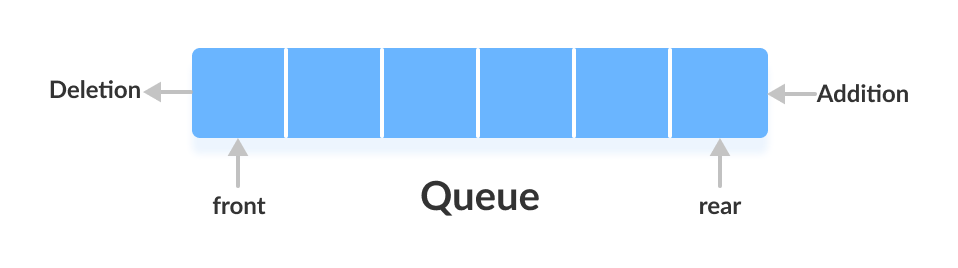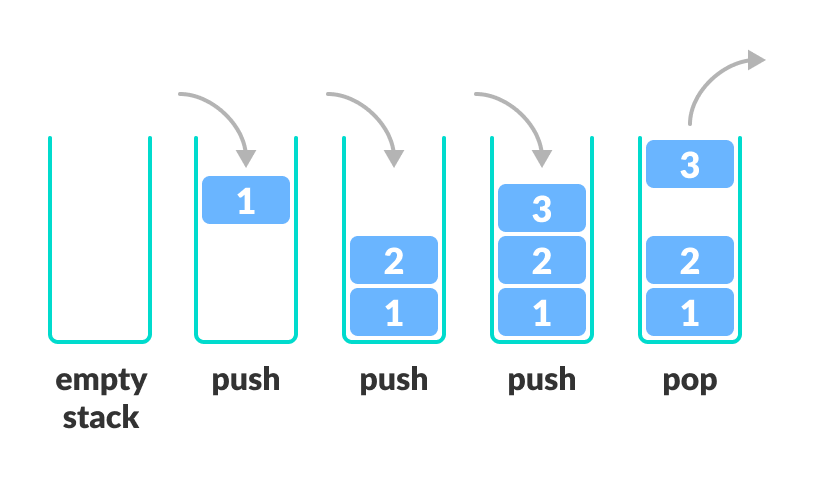abhinabk/python-graph-algorithms - Jovian
Learn data science and machine learning by building real-world projects on Jovian

Graph Algorithms (BFS, DFS, Shortest Paths) using Python

Data Structures and Algorithms in Python is beginner-friendly introduction to common data structures (linked lists, stacks, queues, graphs) and algorithms (search, sorting, recursion, dynamic programming) in Python, designed to help you prepare for coding interviews and assessments.

Ask questions, get help & participate in discussions on the course community forum. Earn a verified certificate of accomplishment for this course by signing up here: http://pythondsa.com .

How to Run the Code

The best way to learn the material is to execute the code and experiment with it yourself. This tutorial is an executable Jupyter notebook. You can run this tutorial and experiment with the code examples in a couple of ways: using free online resources (recommended) or on your computer.

Option 1: Running using free online resources (1-click, recommended)

The easiest way to start executing the code is to click the Run button at the top of this page and select Run on Binder. You can also select "Run on Colab" or "Run on Kaggle", but you'll need to create an account on Google Colab or Kaggle to use these platforms.

Option 2: Running on your computer locally

To run the code on your computer locally, you'll need to set up Python, download the notebook and install the required libraries. We recommend using the Conda distribution of Python. Click the Run button at the top of this page, select the Run Locally option, and follow the instructions.

Graphs in the Real World

Railway networkFlight routesGraph Data StrucutreQuestion: Create a class to represent a graph as an adjacency list in Python

Question: Write a function to add an edge to a graph represented as an adjacency list.

Question: Write a function to remove an edge from a graph represented as a adjacency list.

import jovian
jovian.commit()
[jovian] Attempting to save notebook.. [jovian] Updating notebook "aakashns/python-graph-algorithms" on https://jovian.ai/ [jovian] Uploading notebook.. [jovian] Capturing environment.. [jovian] Committed successfully! https://jovian.ai/aakashns/python-graph-algorithmsQuestion: Represent a graph as an adjacency matrix in Python

Graph Traversal

A real-world graph:Breadth-fist search tree (starting from Frankfurt):Question: Implement breadth-first search given a source node in a graph using Python.BFS pseudocode (Wikipedia):

1  procedure BFS(G, root) is
2      let Q be a queue
3      label root as discovered
4      Q.enqueue(root)
5      while Q is not empty do
6          v := Q.dequeue()
7          if v is the goal then
8              return v
9          for all edges from v to w in G.adjacentEdges(v) do
10              if w is not labeled as discovered then
11                  label w as discovered
12                  Q.enqueue(w)import jovian
jovian.commit()
[jovian] Attempting to save notebook.. [jovian] Updating notebook "aakashns/python-graph-algorithms" on https://jovian.ai/ [jovian] Uploading notebook.. [jovian] Capturing environment.. [jovian] Committed successfully! https://jovian.ai/aakashns/python-graph-algorithms

Question: Write a program to check if all the nodes in a graph are connectednum_nodes3 = 9
edges3 = [(0, 1), (0, 3), (1, 2), (2, 3), (4, 5), (4, 6), (5, 6), (7, 8)]
num_nodes3, len(edges3)
(9, 8)

Depth-first searchQuestion: Implement depth first search from a given node in a graph using Python.DFS pseudocode (Wikipedia):

procedure DFS_iterative(G, v) is
let S be a stack
S.push(v)
while S is not empty do
v = S.pop()
if v is not labeled as discovered then
label v as discovered
for all edges from v to w in G.adjacentEdges(v) do
S.push(w)import jovian
jovian.commit()
[jovian] Attempting to save notebook.. [jovian] Updating notebook "aakashns/python-graph-algorithms" on https://jovian.ai/ [jovian] Uploading notebook.. [jovian] Capturing environment.. [jovian] Committed successfully! https://jovian.ai/aakashns/python-graph-algorithms

Question: Write a function to detect a cycle in a graphWeighted Graphs# Graph with weights
num_nodes5 = 9
edges5 = [(0, 1, 3), (0, 3, 2), (0, 8, 4), (1, 7, 4), (2, 7, 2), (2, 3, 6),
(2, 5, 1), (3, 4, 1), (4, 8, 8), (5, 6, 8)]

num_nodes5, len(edges5)
(9, 10)

Directed Graphsnum_nodes6 = 5
edges6 = [(0, 1), (1, 2), (2, 3), (2, 4), (4, 2), (3, 0)]
num_nodes6, len(edges6)
(5, 6)

Question: Define a class to represent weighted and directed graphs in Python.

import jovian
jovian.commit()
[jovian] Attempting to save notebook.. [jovian] Updating notebook "aakashns/python-graph-algorithms" on https://jovian.ai/ [jovian] Uploading notebook.. [jovian] Capturing environment.. [jovian] Committed successfully! https://jovian.ai/aakashns/python-graph-algorithms

Shortest Paths

Question: Write a function to find the length of the shortest path between two nodes in a weighted directed graph.Dijkstra's algorithm (Wikipedia):1. Mark all nodes unvisited. Create a set of all the unvisited nodes called the unvisited set.
2. Assign to every node a tentative distance value: set it to zero for our initial node and to infinity for all other nodes. Set the initial node as current.
3. For the current node, consider all of its unvisited neighbours and calculate their tentative distances through the current node. Compare the newly calculated tentative distance to the current assigned value and assign the smaller one. For example, if the current node A is marked with a distance of 6, and the edge connecting it with a neighbour B has length 2, then the distance to B through A will be 6 + 2 = 8. If B was previously marked with a distance greater than 8 then change it to 8. Otherwise, the current value will be kept.
4. When we are done considering all of the unvisited neighbours of the current node, mark the current node as visited and remove it from the unvisited set. A visited node will never be checked again.
5. If the destination node has been marked visited (when planning a route between two specific nodes) or if the smallest tentative distance among the nodes in the unvisited set is infinity (when planning a complete traversal; occurs when there is no connection between the initial node and remaining unvisited nodes), then stop. The algorithm has finished.
6. Otherwise, select the unvisited node that is marked with the smallest tentative distance, set it as the new "current node", and go back to step 3.
def update_distances(graph, current, distance, parent=None):
"""Update the distances of the current node's neighbors"""
neighbors = graph.data[current]
weights = graph.weight[current]
for i, node in enumerate(neighbors):
weight = weights[i]
if distance[current] + weight < distance[node]:
distance[node] = distance[current] + weight
if parent:
parent[node] = current

def pick_next_node(distance, visited):
"""Pick the next univisited node at the smallest distance"""
min_distance = float('inf')
min_node = None
for node in range(len(distance)):
if not visited[node] and distance[node] < min_distance:
min_node = node
min_distance = distance[node]
return min_node

num_nodes7 = 6
edges7 = [(0, 1, 4), (0, 2, 2), (1, 2, 5), (1, 3, 10), (2, 4, 3), (4, 3, 4), (3, 5, 11)]
num_nodes7, len(edges7)
(6, 7)

import jovian
jovian.commit()
[jovian] Attempting to save notebook.. [jovian] Updating notebook "aakashns/python-graph-algorithms" on https://jovian.ai/ [jovian] Uploading notebook.. [jovian] Capturing environment.. [jovian] Committed successfully! https://jovian.ai/aakashns/python-graph-algorithms

Binary Heap

A data structure to maintain the running minimum/maximum of a set of numbers, supporting efficient addition/removal.Heap operations:

• Insertion - \(O(log N)\)
• Min/Max - \(O(1)\) (depending on type of heap)
• Deletion - \(O(log N)\)
• Convert a list to a heap - \(O(n)\)

Python's built-in heap: https://docs.python.org/3/library/heapq.html

Question: Implement Dijkstra's shortest path algorithm using the heap module from Python. What is the complexity of the algorithm?

More Problems

Solve more graph problems here: https://leetcode.com/tag/graph/

import jovian
jovian.commit()
[jovian] Attempting to save notebook.. [jovian] Updating notebook "aakashns/python-graph-algorithms" on https://jovian.ai/ [jovian] Uploading notebook.. [jovian] Capturing environment.. [jovian] Committed successfully! https://jovian.ai/aakashns/python-graph-algorithms

SolutionsInput Data

num_nodes1 = 5
edges1 = [(0, 1), (1, 2), (2, 3), (3, 4), (4, 0), (1, 4), (1, 3)]
num_nodes1, len(edges1)
(5, 7)
num_nodes3 = 9
edges3 = [(0, 1), (0, 3), (1, 2), (2, 3), (4, 5), (4, 6), (5, 6), (7, 8)]
num_nodes3, len(edges3)
(9, 8)
num_nodes5 = 9
edges5 = [(0, 1, 3), (0, 3, 2), (0, 8, 4), (1, 7, 4), (2, 7, 2), (2, 3, 6),
(2, 5, 1), (3, 4, 1), (4, 8, 8), (5, 6, 8)]

num_nodes5, len(edges5)
(9, 10)
# Directed graph
num_nodes6 = 5
edges6 = [(0, 1), (1, 2), (2, 3), (2, 4), (4, 2), (3, 0)]
num_nodes6, len(edges6)
(5, 6)
num_nodes7 = 6
edges7 = [(0, 1, 4), (0, 2, 2), (1, 2, 5), (1, 3, 10), (2, 4, 3), (4, 3, 4), (3, 5, 11)]
num_nodes7, len(edges7)
(6, 7)

class Graph:
def __init__(self, num_nodes, edges):
self.data = [[] for _ in range(num_nodes)]
for v1, v2 in edges:
self.data[v1].append(v2)
self.data[v2].append(v1)

def __repr__(self):
return "\n".join(["{} : {}".format(i, neighbors) for (i, neighbors) in enumerate(self.data)])

def __str__(self):
return repr(self)
g1 = Graph(num_nodes1, edges1)
g1
0 : [1, 4]
1 : [0, 2, 4, 3]
2 : [1, 3]
3 : [2, 4, 1]
4 : [3, 0, 1]

Complexity \(O(m + n)\)

def bfs(graph, source):
visited = [False] * len(graph.data)
queue = []

visited[source] = True
queue.append(source)
i = 0

while i < len(queue):
for v in graph.data[queue[i]]:
if not visited[v]:
visited[v] = True
queue.append(v)
i += 1

return queue
bfs(g1, 3)
[3, 2, 4, 1, 0]

Depth First Search

def dfs(graph, source):
visited = [False] * len(graph.data)
stack = [source]
result = []

while len(stack) > 0:
current = stack.pop()
if not visited[current]:
result.append(current)
visited[current] = True
for v in graph.data[current]:
stack.append(v)

return result
dfs(g1, 0)
[0, 4, 1, 3, 2]

Directed and Weighted Graph

class Graph:
def __init__(self, num_nodes, edges, directed=False):
self.data = [[] for _ in range(num_nodes)]
self.weight = [[] for _ in range(num_nodes)]

self.directed = directed
self.weighted = len(edges) > 0 and len(edges) == 3

for e in edges:
self.data[e].append(e)
if self.weighted:
self.weight[e].append(e)

if not directed:
self.data[e].append(e)
if self.weighted:
self.data[e].append(e)

def __repr__(self):
result = ""
for i in range(len(self.data)):
pairs = list(zip(self.data[i], self.weight[i]))
result += "{}: {}\n".format(i, pairs)
return result

def __str__(self):
return repr(self)
g7 = Graph(num_nodes7, edges7, directed=True)
g7
0: [(1, 4), (2, 2)]
1: [(2, 5), (3, 10)]
2: [(4, 3)]
3: [(5, 11)]
4: [(3, 4)]
5: []
g7.weight
[2, 10, 3, 11, 4, []]

Shortest Path - Dijkstra's Algorithm

def update_distances(graph, current, distance, parent=None):
"""Update the distances of the current node's neighbors"""
neighbors = graph.data[current]
weights = graph.weight[current]
for i, node in enumerate(neighbors):
weight = weights[i]
if distance[current] + weight < distance[node]:
distance[node] = distance[current] + weight
if parent:
parent[node] = current

def pick_next_node(distance, visited):
"""Pick the next univisited node at the smallest distance"""
min_distance = float('inf')
min_node = None
for node in range(len(distance)):
if not visited[node] and distance[node] < min_distance:
min_node = node
min_distance = distance[node]
return min_node

def shortest_path(graph, source, dest):
"""Find the length of the shortest path between source and destination"""
visited = [False] * len(graph.data)
distance = [float('inf')] * len(graph.data)
parent = [None] * len(graph.data)
queue = []
idx = 0

queue.append(source)
distance[source] = 0
visited[source] = True

while idx < len(queue) and not visited[dest]:
current = queue[idx]
update_distances(graph, current, distance, parent)

next_node = pick_next_node(distance, visited)
if next_node is not None:
visited[next_node] = True
queue.append(next_node)
idx += 1

return distance[dest], distance, parentshortest_path(g7, 0, 5)
(20, [0, 4, 2, 9, 5, 20], [None, 0, 0, 4, 2, 3])

import jovian
jovian.commit()
[jovian] Attempting to save notebook..